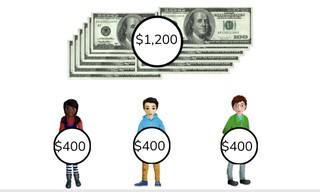Dividing a number greater than 1,000 by a number <10

# Dividing a number greater than 1,000 by a number <10

Students learn to divide a number greater than 1,000 by a number less than 10.8,000 schools use Gynzy92,000 teachers use Gynzy1,600,000 students use Gynzy

## General

The students learn to divide a number greater than 1,000 by a number less than 10.

## Standards

CCSS.Math.Content.4.NBT.B.6

## Learning objective

Students will be able to divide a number greater than 1,000 by a number less than 10 without remainder.

## Introduction

The students solve the division problems with numbers to 1,000.

## Instruction

Explain that in division problems with a number greater than 1,000 you first see what number from the table of the divisor is the closest to but less than the dividend. For the problem 9936 ÷ 3 you are looking for the number that takes the largest number away from the dividend. 3 × 3 = 9. So 3,000 × 3 = 9,000. Subtract 9,000 from 9,936. Now you still have 936 left over. Now you also know that 300 × 3 = 900. Now solve the problem 936 - 900 = 36. Next you do 12 × 3 = 36, 36 - 36 = 0. Now you add 3,000, 300 and 12 together for your answer (3312). Have the students solve the division problems on their own. Then walk them through the steps of solving a story problem.

Check whether the students can divide a number greater than 1,000 by asking the following question:
- What steps do you follow to solve the problem 9,999 ÷ 9?

## Quiz

The students test their understanding of dividing a number greater than 1,000 by a number less than 10 through ten exercises. For some of the exercises the students must choose from possible answers to a division problem, and for others they must provide the answer on their own. Some of the exercises are story problems.

## Closing

Discuss once again the importance of being able to divide a number greater than 1,000 by a number less than 10. As a closing activity the students can drag the money to visualize the given division problems.

## Teaching tips

Have students that have difficulty dividing a number greater than 1,000 first practice with the division tables and dividing a number to 1,000.

### The online teaching platform for interactive whiteboards and displays in schools

• Save time building lessons

• Manage the classroom more efficiently

• Increase student engagement